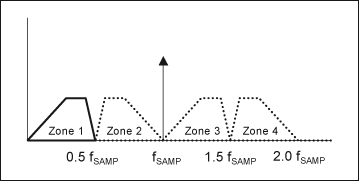# Folded-Frequency Calculator

### Abstract

The following application note provides a fast, easy-to-use tool to determine true and aliased frequency locations of image signals and harmonics in a typical frequency spectrum. Such data is used to analyze the dynamic performance of analog-to-digital (ADC) and digital-to-analog (DAC) converters. The calculator tool was created in Excel and can be downloaded through the link provided in the application note.

This Excel®-based, easy-to-use, Folded-Frequency Calculator provides a quick way to locate integral harmonics of the fundamental frequency in the first Nyquist zone of a sampled data system. The calculator is independent of the sampling process and works for Nyquist-, over-, and undersampling. This tool is useful for users interested in locating various harmonics in the first Nyquist zone of folded frequency spectrum of ADCs and DACs.

This application note discusses the algorithm used for the calculation of folded-frequency locations in the first Nyquist zone, and includes a step-by-step guide for the Folded-Frequency Calculator. Additionally, to lend a deeper insight, this application note briefly discusses the concepts of aliasing and Nyquist in sampled data systems in general, and in data converters in particular.

### Aliasing and Nyquist

Aliasing in a sampled data system is a well-known phenomenon. Aliasing occurs whenever a signal is sampled at a rate below its Nyquist rate, i.e. twice the signal frequency bandwidth. A real-world signal-frequency spectrum contains harmonics of the fundamental frequency, as well as in-band and out-of-band noise frequencies. Inherent device and sampling process nonlinearities manifest themselves in the form of harmonics of the desired fundamental frequency in the output waveform. Any higher order harmonics with frequencies greater than fSAMP/2, with fSAMP being the sampling frequency, fold back into the first Nyquist zone (Figures 1a and 1b) due to aliasing.The fast Fourier transform (FFT) frequency spectrum of a discrete time signal can be divided into an infinite number of fSAMP/2 frequency bands, also known as Nyquist zones. The frequency spectrum between DC and fSAMP/2 is known as the first Nyquist zone. The frequency spectrum repeats itself over different Nyquist zones. Note that the even Nyquist zones appear as mirror images of the odd Nyquist zones (Figure 2).### Aliasing in ADCs and DACs

Aliasing in ADCs is a ramification of the track-and-hold (T/H) processing of the analog signal at the input stage. In the digital signal processing (DSP) domain, T/H processing is equivalent to convolution of the frequency spectrum of the impulse train (due to the sampling clock) with the frequency spectrum of the analog input. This convolution results in periodicity of the frequency spectrum that is observed over various Nyquist zones, as previously explained. When the input signal contains frequency components above the Nyquist frequency (fSAMP/2), the adjacent Nyquist zones start overlapping and result in aliasing.

Aliasing in DACs is a consequence of the zero-order-hold (ZOH) processing (used to avoid code-dependent output glitches) of the discrete time samples at the output stage. ZOH processing in the DSP domain is equivalent to convolution of the sin(x)/x type of frequency spectrum (of the rectangular function appearing due to holding discrete time samples) with the DAC core's output-impulse train frequency spectrum (of varying amplitude, in general). As in the case of ADCs, the periodicity of the output spectrum over different Nyquist zones can be attributed to this convolution.

### Calculator

Mathematically, any frequency component below fSAMP/2 will be present in the frequency spectrum without aliasing. However, due to aliasing, any harmonic component (fHARM) above fSAMP/2 will also appear as an image frequency at |± K x fSAMP ± fHARM |, where K = 1, 2, 3, and so on.

The following algorithm can be used to locate various harmonics in the first Nyquist zone:

```fNYQ = fSAMP/2;
fHARM = N x fFUND; //N is an integer
If (fHARM lies in an odd Nyquist zone) then
fLOC = fHARM % fFUND; //% is the modulus operator
else
fLOC = fFUND - (fHARM % fFUND);
End;
```

where: fNYQ is the Nyquist frequency, fSAMP is the sampling frequency, fFUND is the fundamental frequency of the signal, fHARM is a harmonic frequency of the signal, and fLOC is the location of a harmonic in the first Nyquist zone.

Finding the location (fLOC) of various harmonic frequencies (fHARM) using a simple electronic calculator may require certain iterations. An Excel spreadsheet titled "Folded-Frequency Calculator" is available for download to simplify the process.

The Folded-Frequency Calculator requires two input variables: the sampling frequency (fSAMP) and the fundamental frequency of the signal (fFUND). Using these two variables, the Folded-Frequency Calculator calculates the value of Nyquist frequency (fNYQ), absolute values of various harmonic frequencies (fHARM), and locations of various harmonics in the first Nyquist zone of a folded-frequency spectrum. Table 1 shows an example of a folded-frequency calculation.

 N fNYQ (MHZ) fHARM (MHz) fLOC (MHz) 1 250.000000 29.96826172 29.96826172 2 59.93652344 59.93652344 3 89.90478515 89.90478515 4 119.8730469 119.8730469 5 149.8413086 149.8413086 6 179.8095703 179.8095703 7 209.777832 209.777832 8 239.7460937 239.7460937 9 269.7143555 230.2856445 10 299.6826172 200.3173828 11 329.6508789 170.3491211 12 359.6191406 140.3808594 13 389.5874023 110.4125977 14 419.5556641 80.44433595 15 449.5239258 50.47607423